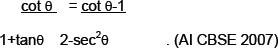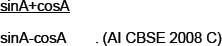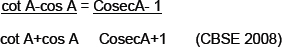# Introduction to Trigonometry-Sample Questions

INTRODUCTION TO TRIGONOMETRY

PREVIOUS YEAR QUESTIONS
1.   Prove that:2.   sin 3θ= cos(θ-6°) and 3θ and θ-6° are acute angles, find value of θ. . (AI CBSE 2008)
3.   If tan A=4/3, then find value of4.   If tan A=b/a, where a and b are real numbers, find value of sin2A .(AI CBSE 2007)
5.   Prove that:6.   If cosθ+sin θ=√2cosθ, show that cosθ-sinθ=√2sinθ . (AI CBSE 2007)
7.   Prove that Sinθ (1+tanθ)+cosθ (1+cotθ)=secθ+cosecθ . . (AI CBSE 2008)
8.   Prove that: (sinθ+cosecθ)2+ (cosθ+secθ)2= 7+tan2θ+cot2θ . (AI CBSE 2008)
9.   If 7 sin2θ+ cos2θ=44, show that tan=1/√3 (AI CBSE 2008)
10.   If sec4A=cosec(A-20°), where 4A is a acute angle, find the value of A. (CBSE 2008)